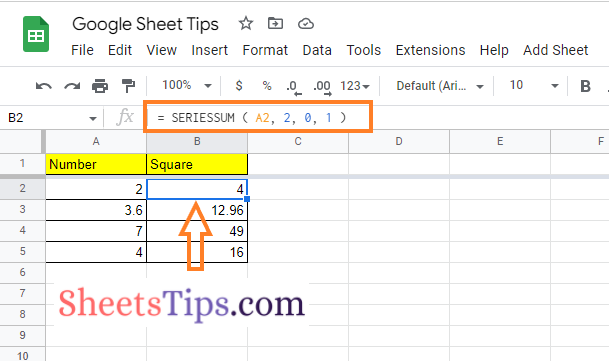# Square Numbers In Google Sheets – How To Square a Number in Google Sheets?

If you are a person who is working with engineering and mathematical calculations, then it is quite common for you to have square numbers. Calculation of square numbers is made easy in Google Sheets. Google Sheets has multiple methods with the help of which one can easily convert a number into square numbers. In this article, let us discuss how to square a number in a spreadsheet using Google Sheets Tips. Read on to find out more.

## How Do You Square Numbers in Google Sheets?

Squaring a number is very easy in Google Sheets. With the help of built-in functions and Apps Script, one can easily find the square of a number. However, before we discuss advanced methods to find the square of a number, let us learn the straight-forward method with the help of which we can find the square of the number by going through the steps below:

• 2nd Step: On the homepage, move to the cell where you want to find the square of the number.
• 3rd Step: Enter the formula “A2*A2“. Here, A2 is the cell range.
• 4th Step: Press the “Return” key and you will find the results as shown in the image given below.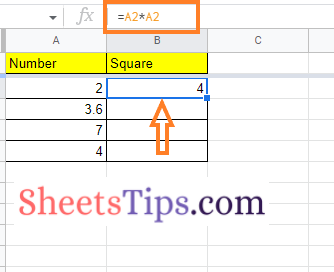### Finding the Square of a Number using the Square Symbol in Google Sheets

Follow the steps as outlined below to find the square of a number using the square symbol in Google Sheets:

• 2nd Step: On the homepage, move to the cell where you want to display the result of the square number.
• 3rd Step: Now enter the formula “A2^2“.
• 4th Step: Press the “Enter” key and you will find the results as shown in the image given below.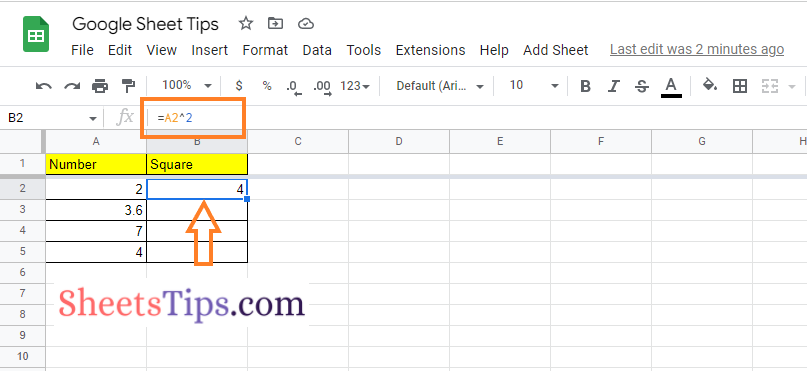### Squaring a Number using the POWER Function in Google Sheets

One can also square a number in Google Sheets using the POWER function. The detailed steps on how to use the POWER function to find the square of the number in the spreadsheet are given below:

• 2nd Step: On the homepage, move to the cell where you want to show the result for the square of a number.
• 3rd Step: Enter the formula “=POWER (A2, 2)“.
• 4th Step: Press the “Enter” key and you will find the results as shown in the image given below.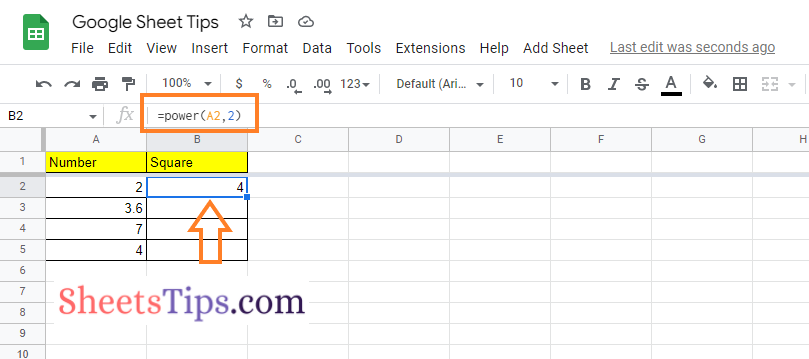### Find the Square of a Number using the SUMSQ Function

Follow the steps given below to find the square of the number using the SUMSQ function. The detailed steps on how to do this in Google Sheets are given below:

• 2nd Step: On the homepage, go to the cell where you want to show the results for the square of the number.
• 3rd Step: Enter the formula “=SUMSQ (A2)“. Here, A2 is the cell range.
• 4th Step: Press the Enter key and you will find the results as shown in the image given below.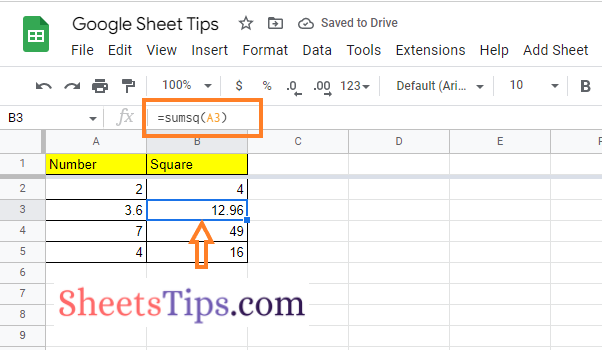### Finding the Square of a Number using the SERIESSUM Function

Another easy method to find the square of the number is to use the SERIESSUM function in Google Sheets. The SERIESSUM function is similar to the SUMSQ function. You can actually find the sum of any mathematical series using this more flexible method. The detailed steps on how to do this in Google Sheets are given below: